## 0x2：什幺是过拟合？

### 1. 判断发生过拟合的现象 – 训练集上得到的模型无法适应测试集

1）数据集2）选用复杂函数进行拟合3） 选用简单函数进行拟合### 2. 过拟合产生的本质原因

```1. 训练集和测试机特征分布不一致：

2. 在有限的样本中搜索过大的模型空间

3. 训练过程中函数过多吸收了噪音数据的影响
fit model的时候加的parameter太多了，导致model太精准地抓住了这组数据的所有variance，不管是主要的数据趋势带来的variance还是噪音带来的variance都一并被拟合在了模型里。

## 0x3：复杂函数一定不好吗？复杂函数一定会导致过拟合吗？

### 2. 故事背后的意义

```第一，判断两个解释哪个才是真正的「简单」是一个非常微妙的事情；

## 0x4：解决过拟合的一个有效的方法 – 正则化

```1. 经典的 bias-variance decomposition；
2. PAC-learning 泛化界解释；
3. Bayes先验解释，这种解释把正则化变成先验```

1. 正则化作用一 – 减少权值参数个数2. 正则化作用二 – 降低权值参数数值

```https://www.jianshu.com/p/1aafbdf9faa6
https://hit-scir.gitbooks.io/neural-networks-and-deep-learning-zh_cn/content/chap3/c3s5ss2.html
https://www.zhihu.com/question/32246256
https://www.zhihu.com/question/20700829```

## 0x1：正则化简介

L1正则化和L2正则化可以看做是 损失函数的惩罚项 。

## 0x2： Lasso回归 – 包含L1正则化的线性回归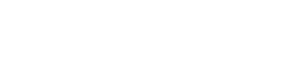，后面一项 α | | w | | 1 即为L1正则化项。 | | w | | 1 是指权值向量 w 中各个元素的 绝对值之和 。

### 1. L1正则化的作用

L1正则化可以产生 稀疏权值矩阵 ，即产生一个稀疏模型，可以用于特征选择。

### 2. 以二维损失函数可视化解释 L1正则化是如何影响模型权重分配的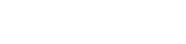，则 J = J0 + L，此时我们的任务变成在 L 约束下求出 J0 取最小值的解。```α 越小，L 的图形越大（上图中的黑色方框）；
α 越大，L 的图形就越小，可以小到黑色方框只超出原点范围一点点；```

```1. 使得权重向量 w 尽量稀疏，即被选中的特征尽量少。且 ；
2. 即使被选中，也有能力尽量使得 w 尽量小；```

### 3. L1正则化的惩罚因子参数怎幺选择

α越大，越容易使得权值向量 w 取得稀疏情况，同时 权值向量 w 值也越小。

## 0x3： Ridge回归 – 包含L2正则化的线性回归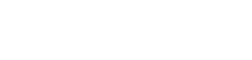，式中加号后面一项 α 即为L2正则化项。 | | w | | 2 是指权值向量 w 中各个元素的 平方和然后再求平方根 。

### 1. L2正则化的作用

L2正则化可以防止模型过拟合（overfitting）。但是这不是L2正则化的专利，L1正则化也能一定程度上防止模型过拟合。

### 2. 以二维损失函数可视化解释 L2正则化是如何使模型权重分配趋向于小值```与未添加L2正则化的迭代公式相比，每一次迭代，θj 都要先乘以一个小于 1 的因子，从而使得 θj 不断减小，因此总得来看，θ是不断减小的；

### 3. L2正则化的惩罚因子参数怎幺选择

λ越大，L2惩罚力度就越大，参数被小值化压制的程度也越大。

## 0x4：L1、L2正则化各自适合的场景

```1. ridge regression（L2） 并不具有产生稀疏解的能力，也就是说参数并不会真出现很多零。假设我们的预测结果与两个特征相关，L2正则倾向于综合两者的影响，给影响大的特征赋予高的权重；
2. 而 L1 正则倾向于选择影响较大的参数，而舍弃掉影响较小的那个；```

## 0x5：不同惩罚参数下，正则化效果可视化

```# -*- coding: utf-8 -*-
from __future__ import print_function
import keras
from keras.datasets import mnist
from keras.models import Sequential, Model
from keras.layers import Dense, Dropout, Flatten, Input
from keras.layers import Conv2D, MaxPooling2D
from keras import backend as K
from keras import initializers
import numpy as np
import matplotlib.pyplot as plt
from keras import regularizers
(x_train, y_train), (x_test, y_test) = mnist.load_data()
x_train = x_train.reshape(x_train.shape, 28, 28, 1)
x_test = x_test.reshape(x_test.shape, 28, 28, 1)
x_train = x_train.astype('float32')
x_test = x_test.astype('float32')
x_train /= 255.0
x_test /= 255.0
y_train = keras.utils.to_categorical(y_train, 10)
y_test = keras.utils.to_categorical(y_test, 10)

def my_reg(weight_matrix):
#return 0    # 无正则化
return 1.0 * K.sum(K.abs(weight_matrix))   # L1正则化
#return 2.0 * K.sum(K.abs(weight_matrix))   # L1正则化
#return 1.0 * K.sum(K.pow(K.abs(weight_matrix), 2))   # L2正则化
#return 2.0 * K.sum(K.pow(K.abs(weight_matrix), 2))   # L2正则化
#return 1.0 * K.sum(K.abs(weight_matrix)) + 1.0 * K.sum(K.pow(K.abs(weight_matrix), 2)) # L1-L2混合正则化
#return K.sum(K.pow(K.abs(weight_matrix), 3))   # L3正则化

# 所有权重初始化为均值0，方差0.5的正态分布
init = initializers.random_normal(mean=0, stddev=0.25, seed=42)
input = Input(shape=(28, 28, 1))
conv1 = Conv2D(32, kernel_size=(3, 3), activation='relu', kernel_initializer=init, kernel_regularizer=my_reg)(input)
conv2 = Conv2D(64, (3, 3), activation='relu', kernel_initializer=init, kernel_regularizer=my_reg)(conv1)
pool1 = MaxPooling2D(pool_size=(2, 2))(conv2)
conv3 = Conv2D(128, (3, 3), activation='relu', kernel_initializer=init, kernel_regularizer=my_reg)(pool1)
pool2 = MaxPooling2D(pool_size=(2, 2))(conv3)
flat = Flatten()(pool2)
dense1 = Dense(128, activation='relu', kernel_initializer=init, kernel_regularizer=my_reg)(flat)
output = Dense(10, activation='softmax', kernel_initializer=init, kernel_regularizer=my_reg)(dense1)
model = Model(inputs=input, outputs=output)
model.summary()
for i in range(40):
model.fit(x_train, y_train, batch_size=128, epochs=1, verbose=0, validation_data=(x_test, y_test))  # 每次只训练一轮
score = model.evaluate(x_test, y_test, verbose=0)
weights = model.get_weights()
all_weights = np.zeros([0, ])
for w in weights:
w_flatten = np.reshape(w, [-1])
all_weights = np.concatenate([all_weights, w_flatten], axis=0)
plt.hist(all_weights, bins=100, color="b", normed=True, range=[-1, 1])
print("epoch=" + str(i) + " loss=%.2f ,acc=%.3f" % (score, score))
plt.title("epoch=" + str(i) + " loss=%.2f ,acc=%.3f" % (score, score))
plt.savefig("mnist_model_weights_hist_%d.png" % (i))
plt.clf()```

### 1. 无正则化### 2. L1正则化 – 惩罚因子 = 1e-4### 3. L1正则化 – 惩罚因子 = 1e-3### 4. L2正则化 – 惩罚因子 = 1e-4### 5. L2正则化 – 惩罚因子 = 1e-3### 6. L1-L2混合正则化### 7. L3正则化## 0x5：从不同正则化参数效果图中我们可以得到什幺信息

```1. L1/L2正则化都可以将权重往0的方向压制，但是L1的压制效果更强，L2比较慢一些，相同惩罚参数的情况下，L2要比L1花更久的时间达到相同的压制效果。
2. 在保证准确率的前提下，L1/L2正则化能够省下更多的元素，这就意味着模型能够大大地减少其存储空间（将矩阵变成稀疏矩阵的形式保存）
3. 从L1/L2的函数图像来看:
1) 元素绝对值在[0,1]之间的时候，L1对于元素的惩罚更大，L2的惩罚小，相对的容忍度更大;
2) 元素绝对值在[1，∞]之间的时候，L1对于元素的惩罚更小，L2的惩罚大，相对的容忍度更小
4. 从斜率角度来看:
1) L2正则化的斜率大要在[1，∞]才能发挥其功力，在[0,1]之间，往0赶的能力不是很强;
2) 然而L1在所有地方斜率都一样，相对而言，在[0,1]之间，其往0赶的能力强```

```https://vimsky.com/article/969.html
https://blog.csdn.net/jinping_shi/article/details/52433975
https://blog.csdn.net/yuweiming70/article/details/81513742
https://blog.csdn.net/zouxy09/article/details/24971995
https://blog.csdn.net/yuweiming70/article/details/81513742```

## 3. 从贝叶斯估计的角度看线性模型中的正则化

```其中正则化项对应后验估计中的先验信息；

## 0x1：不包含正则化的原始线性回归### 1. 由最大似然估计(MLE) – 针对不包含正则项的概率分布进行极大似然估计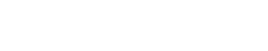## 0x2：引入高斯分布先验的线性回归 – Ridge Regression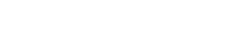。绝对值后面的下标2表示L2范数的意思。

## 0x3：引入拉普拉斯分布(Laplace distribution)先验的线性回归 – LASSO Regression

LASSO – least absolute shrinkage and selection operator.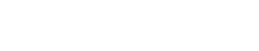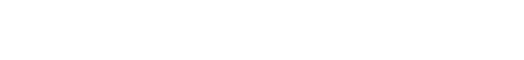。绝对值后面的下标1表示L1范数的意思。

LASSO 仍然是一个 convex optimization（凸优化） 问题，不具有解析解。它的优良性质是能产生

## 0x4：引入L1-L2先验的线性回归 – Elastic Net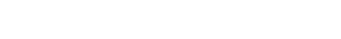。绝对值后面的下标1和下标2表示L1/L2范数的意思。```https://www.cnblogs.com/LittleHann/p/7309511.html#_label3_4_0_2

```https://www.zhihu.com/question/23536142/answer/90135994
https://www.jianshu.com/p/c9bb6f89cfcc
https://blog.csdn.net/zhuxiaodong030/article/details/54408786
http://charleshm.github.io/2016/03/Regularized-Regression/#fn:5

## 4. 从经验风险/结构风险的角度看线性模型中的正则化

```https://www.cnblogs.com/LittleHann/p/7749661.html#_label3_0_2_0

`https://blog.csdn.net/zhihua_oba/article/details/78728880`

## 0x1：决策树中的正则化

`https://www.cnblogs.com/LittleHann/p/7309511.html`